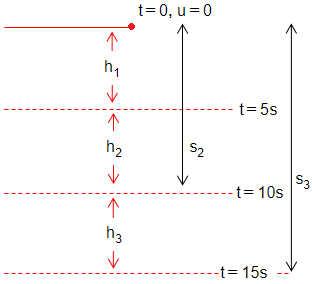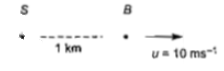# NEET and AIPMT NEET Physics Motion in A Straight Line MCQ Questions SolvedNEET - 2016

Two cars P and Q start from a point at the same time in a straight line and their positions are represented by${x}_{p}\left(t\right)=at+b{t}^{2}$ and ${x}_{Q}\left(t\right)=ft-{t}^{2}$. At what time do the cars have the same velocity?

(a) $\frac{a-f}{1+b}$

(b)$\frac{a+f}{2\left(b-1\right)}$

(c) $\frac{a+f}{2\left(1+b\right)}$

(d) $\frac{f-a}{2\left(1+b\right)}$

Concept Videos :-

#13-Graphical-Meaning-of-Instantaneous
#14-Solved-Examples-7
#12-Instantaneous-Velocity

Concept Questions :-

Instantaneous speed and instantaneous velocity

(d) Velocity of each car is given by

${v}_{p}=\frac{dx\left(t\right)}{dt}=a+2bt$

and ${v}_{Q}=\frac{d{x}_{Q}\left(t\right)}{dt}=f-2t$

It is given that

Difficulty Level:

• 8%
• 15%
• 11%
• 69%
Crack NEET with Online Course - Free Trial (Offer Valid Till September 23, 2019)
NEET - 2016

If the velocity of a particle is v= At+Bt2, where A and B are constants, then the distance travelled by it between 1s and 2s is

(a)3A+7B (b)3/2A+7/3B (c)A/2+B/3 (d)3/2A+4B

Concept Videos :-

#4-Solved Examples 1
#5-Solved-Examples-2
#6-Solved-Examples-3
#7-Solved-Examples-4
#1-Understanding Position
#2-Understanding Path Length
#3-Understanding Displacement

Concept Questions :-

Distance and displacement

(b) Velocity of the particle is given as v=At+Bt where A and B are constants.
=> dx/dt=At+Bt [v=dx/dt]
=> dx=(At+Bt2)dt

Integrating both sides, we get
x2∫dx=2∫(At+Bt2)dt =>Δx=x2-x1=A2∫t dt+B 2∫ t2dt
x1         1                                                 1               1

=A[t2/2]2 +B[t3/3]2
1                1
=A/2(22-12)+B/3(23-13
Distance travelled between 1s and 2s is
Δx=A/2X(3)+B/3(7)=3A/2+7B/3

Difficulty Level:

• 12%
• 68%
• 15%
• 6%
Crack NEET with Online Course - Free Trial (Offer Valid Till September 23, 2019)
NEET - 2015

A particle of unit mass undergoes one-dimensional motion such that its velocity varies according to v(x)=$\beta {x}^{-2n}$ where, $\beta$ and n are constants and x is the position of the particle. The acceleration of the particle as a function of x, is given by

(a) $-2n{\beta }^{2}{x}^{-2n-1}$

(b) $-2n{\beta }^{2}{x}^{-4n-1}$

(c) $-2{\beta }^{2}{x}^{-2n+1}$

(d) $2n{\beta }^{2}{e}^{-4n}$+1

Concept Videos :-

#16-Acceleration
#17-Solved-Examples-9
#18 Solved Examples 10
#19-Solved-Examples-11

Concept Questions :-

Acceleration

$v=\beta {x}^{-2n}\phantom{\rule{0ex}{0ex}}a=v\frac{dv}{dx}=\beta {x}^{-2n}×\beta ×-2n×{x}^{-2n-1}=-2n{\beta }^{2}{x}^{-4n-1}$

Difficulty Level:

• 28%
• 55%
• 11%
• 7%
Crack NEET with Online Course - Free Trial (Offer Valid Till September 23, 2019)
NEET - 2013

A stone falls under gravity. It covers distances h1,h2 and h3 in the first 5 seconds, the next 5 seconds and the next 5 seconds respectively. The relation between h1,h2 and h3 is

(a) h1=2h2=3h3

(b) h1=h2/3=h3/5

(c) h2=3h1 and h3=3h2

(d) h1=h2=h3

Concept Videos :-

#26-Equations-for-Uniform-Acceleration
#27-Equations for Uniform Acceleration 28Calculus Method29
#28-Sign Convention
#29-Solved Examples 16
#31-Solved-Examples-18
#32-Solved Examples 19
#33-Solved Examples 20
#34-Solved-Examples-21
#35 Solved Examples 22
#30-Solved Examples 17

Concept Questions :-

Uniformly accelerated motion

(b)So,

Difficulty Level:

• 14%
• 61%
• 12%
• 15%
Crack NEET with Online Course - Free Trial (Offer Valid Till September 23, 2019)
NEET - 2012

The motion of a particle along a straight line is described by equation

$x=8+12t-{t}^{3}$

where, x is in metre and t in second. The retardation of the particle when its velocity becomes zero,is

(a)24 m${s}^{-2}$                                       (b)zero

(c)6 $m{s}^{-2}$                                         (d)12 $m{s}^{-2}$

Concept Videos :-

#42 Non Uniform Acceleration
#43 Solved Examples 29
#44 Solved Examples 30

Concept Questions :-

Non-uniform acceleration

Given, $x=8+12t-{t}^{3}$

We know $v=\frac{dx}{dt}$

and     $a=\frac{dv}{dt}$

So,     $v=12-3{t}^{2}$

and      a=-6t

At t=2s

At         t=2s

$a=-12m/{s}^{2}$

So, retardation of the particle = 12m/${s}^{2}.$

Difficulty Level:

• 10%
• 21%
• 10%
• 62%
Crack NEET with Online Course - Free Trial (Offer Valid Till September 23, 2019)
NEET - 2011

A boy standing at the top of a tower 20 m height drops a stone. Assuming g=, the velocity with which it hits the ground is

(a) 20 m/s                                         (b) 40 m/s

(c) 5 m/s                                           (d) 10 m/s

Concept Videos :-

#26-Equations-for-Uniform-Acceleration
#27-Equations for Uniform Acceleration 28Calculus Method29
#28-Sign Convention
#29-Solved Examples 16
#31-Solved-Examples-18
#32-Solved Examples 19
#33-Solved Examples 20
#34-Solved-Examples-21
#35 Solved Examples 22
#30-Solved Examples 17

Concept Questions :-

Uniformly accelerated motion

Given,

We have

Difficulty Level:

• 83%
• 8%
• 7%
• 4%
Crack NEET with Online Course - Free Trial (Offer Valid Till September 23, 2019)
NEET - 2011

A particle covers half of its total distance with speed ${\mathrm{v}}_{1}$ and the rest half distance with speed ${\mathrm{v}}_{2}.$ Its average speed during the complete journey is

(a) $\frac{{\mathrm{v}}_{1}{\mathrm{v}}_{2}}{{\mathrm{v}}_{1}+{\mathrm{v}}_{2}}$                                         (b) $\frac{2{\mathrm{v}}_{1}{\mathrm{v}}_{2}}{{\mathrm{v}}_{1}+{\mathrm{v}}_{2}}$

(c) $\frac{2{\mathrm{v}}_{1}^{2}{\mathrm{v}}_{2}^{2}}{{\mathrm{v}}_{1}^{2}+{\mathrm{v}}_{2}^{2}}$                                         (d)  $\frac{{\mathrm{v}}_{1}+{\mathrm{v}}_{2}}{2}$

Concept Videos :-

#8-Average-Speed-26-Average-Velocity
#9-Solved-Examples-5
#10-Solved-Examples-6
#11-Examples-on-Application-of-Graphs
#15-Solved-Examples-8

Concept Questions :-

Average speed and average velocity

Velocity $\mathrm{v}=\frac{\mathrm{s}}{l}⇒\mathrm{s}=\mathrm{vt}$

The average speed of particle

${\mathrm{v}}_{\mathrm{av}}=\frac{\mathrm{s}+\mathrm{s}}{\frac{\mathrm{s}}{{\mathrm{v}}_{1}}+\frac{\mathrm{s}}{{\mathrm{v}}_{2}}}\phantom{\rule{0ex}{0ex}}{\mathrm{v}}_{\mathrm{av}}=\frac{2{\mathrm{v}}_{1}{\mathrm{v}}_{2}}{{\mathrm{v}}_{1}+{\mathrm{v}}_{2}}$

Difficulty Level:

• 9%
• 79%
• 5%
• 9%
Crack NEET with Online Course - Free Trial (Offer Valid Till September 23, 2019)
NEET - 2010

A ball is dropped from a high rise platform at t=0 starting from rest. After 6s another ball is thrown downwards from the same platform with a speed v. The two balls meet at t=18 s. What is the value of v? (take g=10 $m{s}^{-2}$)

(a)                                    (b)

(c)                                    (d)

Concept Videos :-

#45-Relative motion
#46-Relative motion-1
#47-Relative motion-2
#48-Relative motion-3
#49-Relative motion-4
#50-Relative motion-5

Concept Questions :-

Relative motion in 1-D

For first ball, u=0

For second ball, initial velocity =v

${t}_{2}=18-6=12s$

Here,           ${s}_{1}={s}_{2}$

$\frac{1}{2}g{\left(18\right)}^{2}=12v+\frac{1}{2}g{\left(12\right)}^{2}$

Difficulty Level:

• 57%
• 18%
• 15%
• 12%
Crack NEET with Online Course - Free Trial (Offer Valid Till September 23, 2019)
NEET - 2010

A particle moves a distance x in time t according to equation $x={\left(t+5\right)}^{-1}.$The acceleration of particle is proportional to

(a) ${\left(velocity\right)}^{3/2}$                                          (b) ${\left(dis\mathrm{tan}ce\right)}^{2}$

(c) ${\left(dis\mathrm{tan}ce\right)}^{-2}$                                          (d) ${\left(velocity\right)}^{2/3}$

Concept Videos :-

#16-Acceleration
#17-Solved-Examples-9
#18 Solved Examples 10
#19-Solved-Examples-11

Concept Questions :-

Acceleration

Given, distance $x={\left(t+5\right)}^{-1}$                  ...(i)

Differentiating Eq. (i) w.r.t. t, we get

$\frac{dx}{dt}=\left(v\right)=\frac{-1}{{\left(t+5\right)}^{2}}$               ...(ii)

Again, differentiating Eq.(i)w.r.t. t, we get

$\frac{{d}^{2}x}{d{t}^{2}}=\left(a\right)=\frac{2}{{\left(t+5\right)}^{3}}$         ...(iii)

Comparing Eqs.(ii) and(iii),we get

$\left(a\right)\propto {\left(v\right)}^{3/2}$

Difficulty Level:

• 56%
• 17%
• 16%
• 14%
Crack NEET with Online Course - Free Trial (Offer Valid Till September 23, 2019)
NEET - 2009

A bus is moving with a speed of  on a straight road. A scooterist wishes to overtake the bus in . If the bus is at a distance of  from the scooterist, with what minimum speed should the scooterist chase the bus?

(a)                                          (b)

(c)                                          (d)

Concept Videos :-

#45-Relative motion
#46-Relative motion-1
#47-Relative motion-2
#48-Relative motion-3
#49-Relative motion-4
#50-Relative motion-5

Concept Questions :-

Relative motion in 1-D

Let v be the relative velocity of scooter(s) w.r.t bus (B), then

$\mathrm{v}={\mathrm{v}}_{\mathrm{S}}-{\mathrm{v}}_{\mathrm{B}}$Relative velocity=displacement/time

Now, substituting the value of v in Eq. (i), we get

Difficulty Level:

• 59%
• 15%
• 13%
• 15%
Crack NEET with Online Course - Free Trial (Offer Valid Till September 23, 2019)